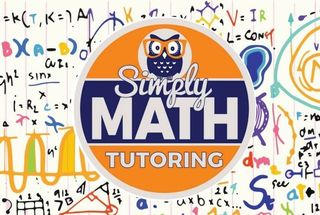\$749.00 - \$749.00

# 5th Grade Math BootCamp – 4 weeks• Ages:

4 week BootCamp Details:

Place values and number sense

→    Place values
→    Convert between place values
→    Compare numbers
→    Word names for numbers
→    Rounding
→    Even or odd: arithmetic rules

→    Add and subtract whole numbers
→    Add and subtract whole numbers: word problems
→    Complete addition and subtraction sentences
→    Fill in the missing digits
→    Choose numbers with a particular sum or difference
Multiplication

→    Multiply by one-digit numbers
→    Multiply by one-digit numbers: word problems
→    Multiplication patterns over increasing place values
→    Multiply numbers ending in zeroes
→    Multiply numbers ending in zeroes: word problems
→    Properties of multiplication
→    Choose numbers with a particular product
Division

→    Division facts to 12
→    Division facts to 12: word problems
→    Divisibility rules
→    Divisibility rules: word problems
→    Divide by one-digit numbers
→    Divide by one-digit numbers: word problems
→    Divide by one-digit numbers: interpret remainders

Number sequences

→    Complete an increasing number sequence
→    Complete a geometric number sequence
→    Use a rule to complete a number sequence
→    Number sequences: word problems
→    Number sequences: mixed review
Probability

→    Understanding probability
→    Find the probability
→    Make predictions
→    Combination